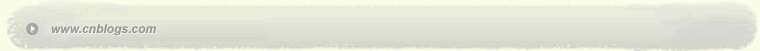C++分析研究 C++

 < 2013年6月 >
2627282930311
2345678
9101112131415
16171819202122
23242526272829
30123456

• 随笔 - 92
• 文章 - 4
• 评论 - 4
• 引用 - 0

# 文章档案

•

### 评论排行榜

m[i+1].w > m[i].w && m[i+1].s < m[i].s

d[i]表示以i为起点的最长路径
dp（i, n）//求解以i为起点的最长路径

[html]
#include <iostream>
using namespace std;
struct Mouse{
int w, s;
Mouse（int _w, int _s）： w（_w）， s（_s） {}
Mouse（）{}
};
Mouse mouse;
int G;
int d;
int dp（int i, int n） {
int& ans = d[i];
if （ans > 0） return ans;
ans = 1;
for （int j = 1; j <= n; j++）
if （G[i][j]） {
int tmp = dp（j, n） + 1;
if （ans < tmp） {
ans = tmp;
}
}
return ans;
}
void print_path（int i, int n） {
cout 《 i 《 endl;
for （int j = 1; j <= n; ++j）
if （G[i][j] && d[i] == d[j] + 1） {
print_path（j, n）；
break;
}
}
int main（） {
int w,s,n = 0;
memset（d, -1, sizeof（d））；
while （scanf（"%d%d", &w, &s） != EOF）
mouse[++n] = Mouse（w, s）；
for （int i = 1; i <= n; ++i） {
for （int j = 1; j <= n; ++j）
if （mouse[j].w > mouse[i].w && mouse[j].s < mouse[i].s）
G[i][j] = 1;
else
G[i][j] = 0;
}
int MAX = 0;
int ans;
for （int i = 1; i <= n; i++）
if （MAX < dp（i, n）） {
MAX = d[i];
ans = i;
}
cout 《 ans 《 endl;
print_path（ans, n）；
return 0;
}

dp[i] = max {dp[j]}+1 && （m[j].w > m[i].w && m[j].s < m[i].s 表示j可以接在j前面 ） j = i+1, … n

[html]
#include <iostream>
using namespace std;
struct Mouse{
int w, s, id, next;
Mouse（int _w, int _s）： w（_w）， s（_s）， next（-1） {}
Mouse（）{}
};
Mouse m;
int dp;
int cmp（const void *a, const void *b） {
if （（（Mouse*）a）->w == （（Mouse*）b）->w）
return （（Mouse*）b）->s - （（Mouse*）a）->s;
else
return （（Mouse*）a）->w - （（Mouse*）b）->w;
}
int main（） {
int w, s, n = 1;
while （cin 》 m[n].w 》 m[n].s）
m[n].id = n++;
--n;
qsort（m+1, n, sizeof（m）， cmp）；
int max = 0;
int flag;
for （int i = n; i >= 1; --i） {
dp[i] = 1;
for （int j = i + 1; j <= n; ++j） {
if （m[j].w > m[i].w && m[j].s < m[i].s）
if （dp[i] < dp[j] + 1） {
dp[i] = dp[j] + 1;
m[i].next = j;
}
}
if （max < dp[i]） {
max = dp[i];
flag = i;
// dp[i]表示以i开头的最大长度
}
}
cout 《 max 《 endl;
for （int i = 1; i <= max; ++i） {
cout 《 m[flag].id 《 endl;
flag = m[flag].next;
}
return 0;
}

posted on 2013-06-25 20:28 HAOSOLA 阅读(253) 评论(0)  编辑 收藏 引用Copyright © HAOSOLA Powered by: 博客园 模板提供：沪江博客